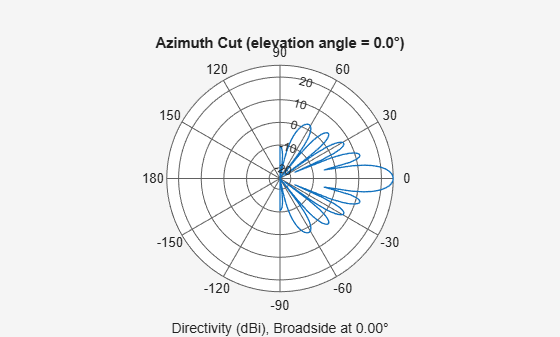# phased.SincAntennaElement

Sinc antenna element

Since R2021b

## Description

The `SincAntennaElement` System object™ models an antenna with a Sinc Response. A sinc antenna is representative of a uniformly illuminated rectangular antenna. Sinc antenna patterns are often used as an approximation for sector or array antennas. The sidelobes are –13.6 dB relative to the main beam gain. The 0° azimuth and 0° elevation is considered to be the main response axis of the antenna. When placed in a linear or a rectangular array, the main response axis is aligned with the array normal.

To compute the response of the antenna element for specified directions:

1. Create the `phased.SincAntennaElement` object and set its properties.

2. Call the object with arguments, as if it were a function.

## Creation

### Syntax

``antenna = phased.SincAntennaElement``
``antenna = phased.SincAntennaElement(Name=Value)``

### Description

````antenna = phased.SincAntennaElement` creates a sinc antenna System object, `antenna`. This object models an antenna element whose response is a sinc antenna, which is representative of a uniformly illuminated rectangular antenna. The sidelobes are –13.6 dB relative to the main beam gain.```

example

````antenna = phased.SincAntennaElement(Name=Value)` creates a sinc antenna object, `antenna`, with each specified property set to the specified value. You can specify multiple name-value arguments in any order. For example, `FrequencyRange=[1e6 1e9]` specifies that the antenna operates in a frequency range from 1 MHz to 1 GHz.```

## Properties

expand all

Unless otherwise indicated, properties are nontunable, which means you cannot change their values after calling the object. Objects lock when you call them, and the `release` function unlocks them.

If a property is tunable, you can change its value at any time.

Operating frequency range of the antenna, specified as a nonnegative, real-valued, 1-by-2 row vector in the form `[LowerBound HigherBound]`. The antenna element has no response outside the specified frequency range. Units are in Hz.

Data Types: `double`

Beamwidth of the antenna pattern, specified as either a scalar or a 1-by-2 real-valued vector. When the specified value is a 1-by-2 vector, it has the form of `[AzimuthBeamwidth ElevationBeamwidth]`. If the specified value is a scalar, the azimuth and elevation beamwidths are equal. Units are in degrees.

Example: `15`

Data Types: `double`

## Usage

### Syntax

``RESP = antenna(FREQ,ANG)``

### Description

example

````RESP = antenna(FREQ,ANG)` returns the antenna voltage response `RESP` at operating frequencies specified in `FREQ` and directions specified in `ANG`.```

### Input Arguments

expand all

Operating frequency of the antenna element, specified as a nonnegative scalar or nonnegative, real-valued 1-by-L row vector. Frequency units are in Hz.

`FREQ` must lie within the range of values specified by the `FrequencyRange` or the `FrequencyVector` property of the element. Otherwise, the element produces no response and the response is returned as `–Inf`. Element objects use the `FrequencyRange` property, except for `phased.CustomAntennaElement`, which uses the `FrequencyVector` property.

Example: `[1e8 2e6]`

Data Types: `double`

Azimuth and elevation angles of the response directions, specified as a real-valued 1-by-M row vector or a real-valued 2-by-M matrix, where M is the number of angular directions. Angle units are in degrees. The azimuth angle must lie in the range –180° to 180°, inclusive. The elevation angle must lie in the range –90° to 90°, inclusive.

• If `ANG` is a 1-by-M vector, each element specifies the azimuth angle of the direction. In this case, the corresponding elevation angle is assumed to be zero.

• If `ANG` is a 2-by-M matrix, each column of the matrix specifies the direction in the form `[azimuth;elevation]`.

The azimuth angle is the angle between the x-axis and the projection of the direction vector onto the xy-plane. This angle is positive when measured from the x-axis toward the y-axis. The elevation angle is the angle between the direction vector and xy-plane. This angle is positive when measured toward the z-axis. See the definition of Azimuth and Elevation Angles.

Example: `[110 125; 15 10]`

Data Types: `double`

### Output Arguments

expand all

Voltage response of the antenna element, returned as a matrix of size M-by-L. In this matrix, M represents the number of angles specified in `ANG` and L represents the number of frequencies specified in `FREQ`.

Data Types: `double`

## Object Functions

To use an object function, specify the System object as the first input argument. For example, to release system resources of a System object named `obj`, use this syntax:

`release(obj)`

expand all

 `directivity` Directivity of antenna or transducer element `beamwidth` Compute and display beamwidth of sensor element pattern `pattern` Plot antenna or transducer element directivity and patterns `patternAzimuth` Plot antenna or transducer element directivity and pattern versus azimuth `patternElevation` Plot antenna or transducer element directivity and pattern versus elevation `isPolarizationCapable` Antenna element polarization capability
 `step` Run System object algorithm `release` Release resources and allow changes to System object property values and input characteristics `reset` Reset internal states of System object

## Examples

collapse all

Create a sinc antenna and plot its azimuth response. The antenna can work between 800 MHz and 1.2 GHz and has an operating frequency of 1 GHz.

```element = phased.SincAntennaElement( ... FrequencyRange=[800e6 1.2e9]); fc = 1e9; pattern(element,fc,-180:180,0,CoordinateSystem="polar")```Find the response of the antenna at the boresight.

```ang = [0 0]'; resp = element(fc,ang)```
```resp = 1 ```